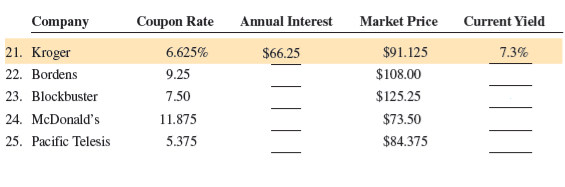/
/
/
Calculate the annual interest and current yield of the following
Not my Question
Flag Content

# Question : Calculate the annual interest and current yield of the following

Calculate the annual interest and current yield of the following bonds.23, 24. Calculate the annual interest and current yield for the following bonds: Note: Refer to these problems in the text for input data. Blockbuster McDonald's Coupon rate = Par value = Annual interest = Market price = Current yield =

## Solution 5 (1 Ratings )

Solved
Mathematics 7 Months Ago 13 Views